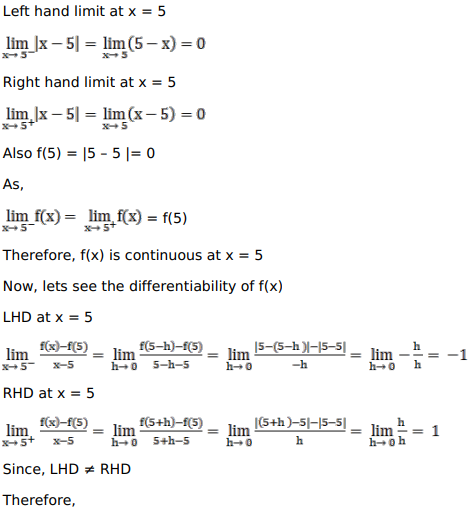# Solve this followingQuestion:

Show that $f(x)=|x-5|$ is continuous but not differentiable at $x=5$

Solution:$f(x)$ is not differentiable at $x=5$